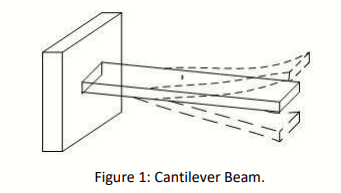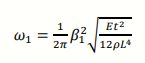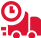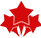# SEMM3023: The determination of the fundamental natural frequency for cantilever beams is crucial in many engineering applications: Applied numerical method Report, UMT, Malaysia

 University Universiti Teknologi Malaysia (UMT) Subject SEMM3023: Applied numerical method

Problem Statement

The determination of the fundamental natural frequency for cantilever beams is crucial in many engineering applications. Here, a cantilever beam refers to a beam that is fixed on one end and free on the other end as shown in Figure 1 below.Generally, a cantilever beam would have an infinite number of natural frequencies corresponding to its vibration modes. However, the fundamental natural frequency refers to the natural frequency of the beam under its first vibration mode. For thin beams, the Euler-Bernoulli beam theory can be used to determine this natural frequency.

The fundamental natural frequency of a cantilever beam is determined by the following equation:where 𝜔1 is the fundamental natural frequency of the beam in Hz, 𝐸 is the beam Young’s modulus, 𝑡 is the thickness of the beam, 𝜌 is the beam’s density, 𝐿 is the length of the beam and 𝛽1 is the fundamental eigenfrequency of the beam which can be determined as the smallest absolute value from the characteristic equation of the following matrix in equation (2). In equation (2), 𝑛 corresponds to the vibration mode number.1. Given that the eigenvalue for matrix [A] in equation (2) is  = −cos (𝛽𝑛).
i) Derive the characteristic equation of matrix [A] in equation (2). Show all your
work when deriving the characteristic equation.
ii) Is it possible to solve for 𝛽𝑛 analytically? If yes, solve it. If no, suggest another method to use to solve for 𝛽𝑛.

2. Based on the characteristic equation derived from Question 1,
i) Suggest four methods you can use to solve for 𝛽𝑛 numerically. Among the four methods you have suggested, are there any methods that may be less favourable to use? If yes, explain why.
ii) Select a numerical method to solve for 𝛽𝑛 and explain in detail and in your own words, all the steps required to implement the chosen method for this problem. (Calculation is NOT required for this question).

3. Using ONLY MATLAB or OCTAVE as your programming platform,
i) Explain the algorithm you will apply in your programming platform to implement the chosen numerical method from Question 2. (NO code is required for this question).
ii) Develop a flowchart based on your algorithm and explain the flowchart. (NO code is required for this question. You may want to refer to your SEMM/SKMM 1013 notes for this question).
iii) Given that 𝐸 = 69 𝐺𝑃𝑎, 𝑡 = 0.1 𝑐𝑚, 𝜌 = 2700 𝑘𝑔𝑚−3 , 𝐿 = 20 𝑐𝑚. Develop a SINGLE script of code in your programming platform to solve for 𝛽𝑛 AND calculate the fundamental natural frequency of the beam, 𝜔1, in Hz to four decimal places. For your initial guess use any values between 0 and 2. For your stopping criteria, stop the iteration when 𝜀 ≤ 0.01. Also, assume that 𝛽𝑛 = 𝛽1. (Please fully comment on your code and display the output of your code in table form as shown in the lecture. Paste your code inside the report. DO NOT screenshot your code for the report. Make sure that your code reflects the flowchart, algorithm, and steps that you have given).

4.

i) Discuss a method to verify your result in Question 3. Hence, verify your results by calculating the percentage error and explaining the significance of the error. (Hint: Solutions from in-built functions in programming software can sometimes be considered as an ‘exact’ solution).
ii) Explain what you learned from the chosen numerical method in Question 2. What improvements can be made in Question 3 to increase the accuracy of your results?

## Get Help By ExpertOnline Exam & Assignment Writing Services51770+ Orders Delivered4.9/5
5 Star Rating

Confidential & Secure Assignment Help For

Group Assignment Help

Online Exam -Test & Quiz

Cheapest Price Quote

Diploma & Certificate Levels

Semester & FYP Papers

Summative & Individual

GBA & Reflective

Last Minute Assistance

##### CSC318 Web Application Development Assignment Sample UITM Malaysia
The assignment sample is designed to teach the basics of web programming. Students are introduced to mark-up language, client-side scripting, and server-side scripting - among other things. Web programming is…
Computer & It
##### TAX317 Taxation 2 UiTM assignment example Malaysia
The taxation of UIIM is one of the most pressing issues in the country today. Several major corporations have been or will be based in Malaysia, and the government has…
Finance

UP TO 15 % DISCOUNT

Instant Paper Writing Services by Native Malaysia Writers

Plagiarism Free Solutions
100% Original Work
24*7 Online Assistance
Native PhD Experts
Hire a Writer Now